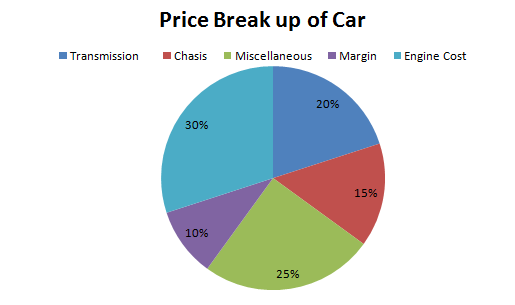Directions: Study the pie charts given below and answer the following questions.The Total Cost Involved in manufacturing a car = Rs. 100000

Question 1: What was the cost of engine?
(a) Rs. 50000
(b) Rs. 60000
(c) Rs. 20000
(d) Rs. 30000

Question 2: What is the cost of Gear Box?
(a) Rs. 9000
(b) Rs. 6000
(c) Rs. 3000
(d) Rs. 15000

Question 3: What percentage of total cost is contributed by the brake?
(a) 55%
(b) 6.6%
(c) 6%
(d) 5.4%

Question 4: If the price of tyres goes up by 25%, by what amount should be the sale price be increased to maintain the amount of profit?
(a) Rs. 750
(b) Rs. 2250
(c) Rs. 3750
(d) Rs 375

Question 5: If transmission cost increases by 20%, by what amount is the profit reduced (total selling price of car remains same)?
(a) Rs. 3000
(b) Rs. 4000
(e) Rs. 6000
(d) Cannot be determined

Question 6: What is the profit percentage?
(a) 10%
(b) 9.09%
(c) 11.11%
(d) Cannot be determined

Question 7: What percentage of cost price is contributed by clutch?
(a) 6%
(b) 2%
(c) 3%
(d) Cannot be determined

Question 8: If transmission cost increases by 10% and Engine cost increases by 20%, what is the percentage contribution of transmission to the cost?
(a) 20%
(b) 20.37%
(c) 21.86%
(d) 21.98%

The engine costs 30% of 100000 = Rs30000

The correct option is (d)

The cost of gear box = 15/100 x 20/100 x 100000 = Rs. 3000

The correct option is (c)

If the total cost is Rs 100, then the cost of Transmission is Rs 20 and of brakes = 30% of 20 = Rs 6

Hence, brakes constitute 6% of the total cost.

The correct option is (c)

Cost of the tyre = 15/100 x 20/100 x 100000 = Rs. 3000

If the cost is increased by 25% the price should be increased by ¼ x 3000 = Rs. 750

The correct option is (a).

The transmission cost is 20% of Rs 100000 = Rs 20000

If the transmission cost is increased by 20% then the new cost will be 20000 × 1.2 = Rs 24000

As the selling price of the car is same as before, so the profit will reduce by 24000 – 20000 = Rs 4000

The correct option is (b)

The total cost of car to manufacturer is given to be Rs 100000 but the selling price is not given.

Hence,the profit percentage cannot be determined.

The cost of clutch is 30/100 x 20/100 x 100000 = Rs. 6000

Hence, the required percentage = (6000/100000) x 100 = 6%

Correct option is (a).

The transmission cost is 20% of 100000 = Rs 20000

The cost of engine = 30% of 100000 = Rs 30000

Now the transmission cost increases by 10%, so new transmission cost = Rs 22000

and similarly, the new engine cost is Rs 36000

Total increase in the cost of car = 2000 + 6000 = Rs 8000

Hence, the new cost of car = Rs 108000

The required percentage = (22000/108000) x 100 = 20.37%

The correct option is (b)

### Want to explore more Data Interpretation Sets?

Get Posts Like This Sent to your Email
Updates for Free Live sessions and offers are sent on mail. Don't worry: we do not send too many emails..:)
Get Posts Like This Sent to your Email
Updates for Free Live sessions and offers are sent on mail. Don't worry: we do not send too many emails..:)